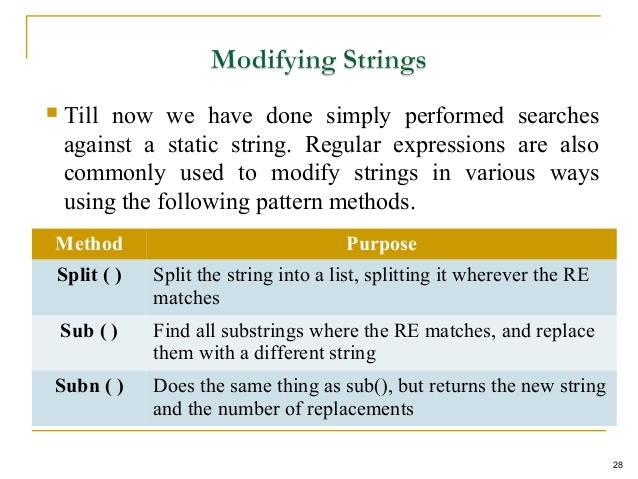/  Technology   /  Python   /  Explain split(), sub(), subn() methods of “re” module in Python.## Explain split(), sub(), subn() methods of “re” module in Python.

The re module in python refers to the module Regular Expressions (RE). It specifies a set of strings or patterns that matches it. Metacharacters are used to understand the analogy of RE.

Function split()

This function splits the string according to the occurrences of a character or a pattern. When it finds that pattern, it returns the remaining characters from the string as part of the resulting list.  The split method should be imported before using it in the program.

Syntax:    re.split (pattern, string, maxsplit=0, flags=0)

Example:

```From re import split

print(split('\W+', 'Words, words , Words'))

print(split('\W+', "Word's words Words"))

print(split('\W+', 'On 12th Jan 2016, at 11:02 AM'))

print(split('\d+', 'On 12th Jan 2016, at 11:02 AM'))

output:

['Words', 'words', 'Words']

['Word', 's', 'words', 'Words']

['On', '12th', 'Jan', '2016', 'at', '11', '02', 'AM']

['On ', 'th Jan ', ', at ', ':', ' AM']```

Function sub()

Syntax:

re.sub (pattern, repl, string, count=0, flags=0)

This function stands for the substring in which a certain regular expression pattern is searched in the given string (3rd parameter). When it finds the substring, the pattern is replaced by repl (2nd parameter). The count checks and maintains the number of times this has occurred.

Example :

```import re

print(re.sub('ub', '~*' , 'Subject has Uber booked already', flags = re.IGNORECASE)) print(re.sub('ub', '~*' , 'Subject has Uber booked already'))

print(re.sub('ub', '~*' , 'Subject has Uber booked already', count=1, flags = re.IGNORECASE))

print(re.sub(r'\sAND\s', ' & ', 'Baked Beans And Spam', flags=re.IGNORECASE))

Output:

Baked Beans & Spam```

Function subn()

Syntax:

re.subn (pattern, repl, string, count=0, flags=0)

This function is similar to sub() in all ways except the way in which it provides the output. It returns a tuple with count of total of all the replacements as well as the new string.

```Example:

import re

print(re.subn('ub', '~*' , 'Subject has Uber booked already'))

t = re.subn('ub', '~*' , 'Subject has Uber booked already', flags = re.IGNORECASE)

print(t)

print(len(t))

print(t)

Output:            ('S~*ject has Uber booked already', 1)

('S~*ject has ~*er booked already', 2)

Length of Tuple is:  2